# String Matching Analysis¶

Consider a string of finite length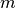Let it be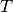. Finding whether a string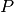of length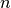exsists inis known as String Matching, Following is some of the comparision based String Matching Algorithms.

• Brute Force String Matching Algorithm
• Horspool String Matching
• Boyer - Moore String Matching

Before looking at the analysis part, we shall examine the Language in built methods to sorting

## The in operator and str.index()¶

We have already seen the in operator in several contexts. Let’s see the working of in operator again

In :

x = 'this is some random text used for illustrative purposes'

In :

x

Out:

'this is some random text used for illustrative purposes'

In :

'this' in x

Out:

True

In :

'not' in x

Out:

False

In :

x.index('is')

Out:

2

In :

x.index('not')

---------------------------------------------------------------------------
ValueError                                Traceback (most recent call last)
<ipython-input-6-a1f052cc5af7> in <module>()
----> 1 x.index('not')



## Standard import statement¶

In :

from openanalysis.string_matching import StringMatchingAlgorithm,StringMatchingAnalyzer


StringMatchingAlgorithm is the base class providing the standards to implement sorting algorithms, SearchVisualizer visualizes and analyses the algorithm

## StringMatchingAlgorithm class¶

Any String Matching Algorithm, which has to be implemented, has to be derived from this class. Now we shall see data members and member functions of this class.

### Data Members¶

• name - Name of the Searching Algorithm
• count - Holds the number of basic operations performed

### Member Functions¶

• __init__(self, name): - Initializes algorithm with a name
• match(self, text, pattern) _ The base String Matching function. Sets count to 0.

## An example …. Horspool String Matching Algorithm¶

Now we shall implement the class Horspool

In :

class Horspool(StringMatchingAlgorithm):                                   # Must derive from StringMatchingAlgorithm
def __init__(self):
StringMatchingAlgorithm.__init__(self, "Hosrpool String Matching")
self.shift_table = {}
self.pattern = ''

def generate_shift_table(self, pattern):                              # class is needed to include helper methods
self.pattern = pattern
for i in range(0, len(pattern) - 1):
self.shift_table.update({pattern[i]: len(pattern) - 1 - i})

def match(self, text: str, pattern: str):
StringMatchingAlgorithm.match(self, text, pattern)
self.generate_shift_table(pattern)
i = len(self.pattern) - 1
while i < len(text):
j = 0
while j < len(self.pattern) and text[i-j] == self.pattern[len(self.pattern)-1-j]:
j += 1
self.count += j                                         # Increment count here
if j == len(self.pattern):
return i-len(self.pattern)+1
if text[i] in self.shift_table:
i += self.shift_table[text[i]]
else:
i += len(self.pattern)
return -1


## StringMatchingAnalyzer class¶

This class provides the visualization and analysis methods. Let’s see its methods in detail

• __init__(self, matching): Initializes visualizer with a String Matching Algorithm.
• searcher is a class, which is derived from StringMatchingAlgorithm
• analyze(self):
• Plots the number of basic operations performed
• Both Text length and Pattern Length are varied
• Samples are chosen randomly from pre defined large data
In :

StringMatchingAnalyzer(Horspool).analyze()

please wait while analysing...Note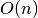performance of algorithm

## Example File¶

You can see more examples at Github